## Excel get or calculate age from birth date

If you have a list of the employee's date of birth in Excel worksheet, now, you want to calculate the age for each of them. In Excel, there are some useful functions, such as YEARFRAC or DATEDIF which can help you to get the age from the birthday quickly and easily.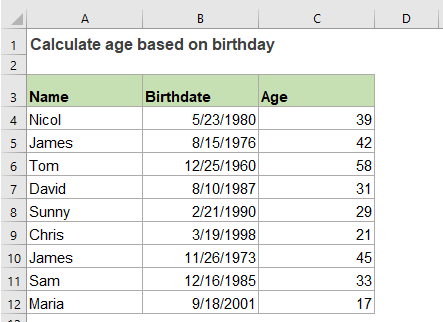#### Calculate age based on date of birth with YEARFRAC function

In Excel, the YEARFRAC function can help you to get the age from the given birth date, the generic syntax is:

=YEARFRAC(birthdate, TODAY())
• birthdate: The date of the birthday.
• TODAY(): This function returns the today's date.

So, please use the below formula into a blank cell:

=YEARFRAC(B2,TODAY())

And then, drag the fill handle down to the cells you want to apply this formula, all the ages have been calculated and displayed as decimal numbers in cells, see screenshot: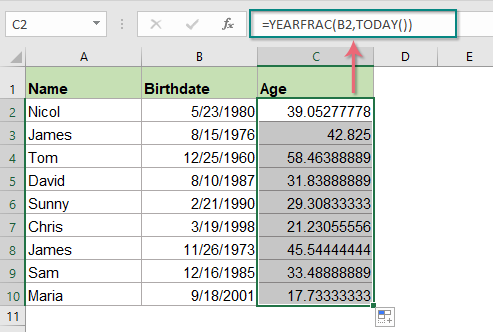Tips: The calculated result is decimal number by using the YEARFRAC function, to make the age number as integer, you should combine the INT function as this:

=INT(YEARFRAC(B2,TODAY()))

And you will get the ages as integers: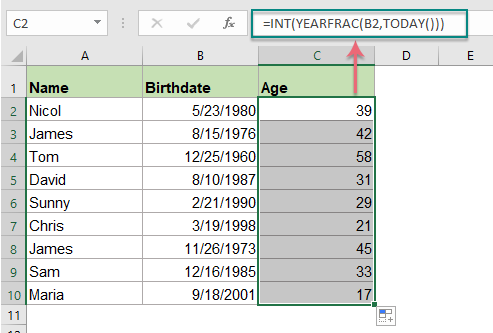#### Calculate age based on date of birth with DATEDIF function

The DATEDIF function also can convert the birth date to age, the generic syntax is:

=DATEDIF(birthdate, TODAY(), "y")
• birthdate: The date of the birthday.
• TODAY(): This function returns the today's date.
• y: It returns the number of complete years from the birth date to current date.

Now, please enter or copy the following formula into a blank cell:

=DATEDIF(B2, TODAY(), "y")

And all the ages have been calculated based on the birth date, see screenshot: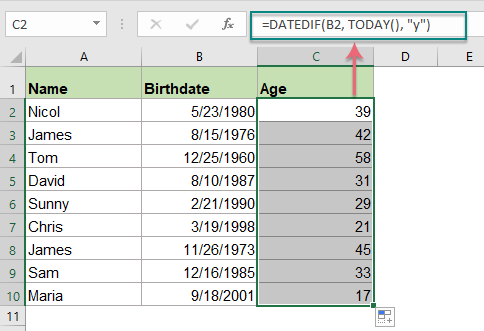#### Calculate age based on date of birth in years, months and days

If you want to get the exact age of the persons’, such as how many years, months and days from their birth dates to current date. You should concatenate the DATEIF functions into one single formula as this:

=DATEDIF(B2,TODAY(),"Y") & " Years, " & DATEDIF(B2,TODAY(),"YM") & " Months, " & DATEDIF(B2,TODAY(),"MD") & " Days"

And then, you will get the results as following screenshot shown: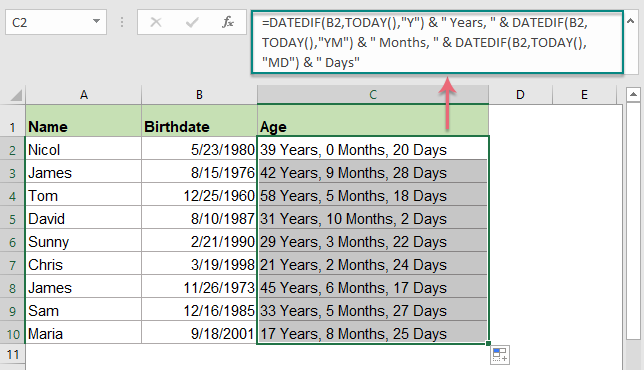Tips: If you want to ignore the 0 year, month or day when applying the above formula, you can combine the IF function to test the 0’s. please use this formula:

=IF(DATEDIF(B2, TODAY(),"y")=0,"",DATEDIF(B2, TODAY(),"y")&" Years, ")& IF(DATEDIF(B2, TODAY(),"ym")=0,"",DATEDIF(B2, TODAY(),"ym")&" Months, ")& IF(DATEDIF(B2, TODAY(),"md")=0,"",DATEDIF(B2, TODAY(),"md")&" Days")

And then, you will get the below screenshot as you need, all ages are displayed in years, months and days without 0 value: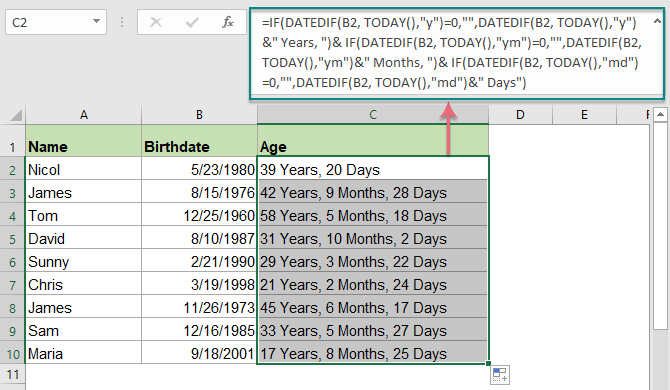#### Calculate age on specific date in Excel

Sometimes, you would like to get the age from birth date to a specific date instead of current date, in this case, you just need to change the TODAY() function in the second argument with the specific date. Such as:

=DATEDIF(birthdate, specific_date, "y")
• birthdate: The date of the birthday.
• specific_date: The end date that you want to calculate the age from the birth date.
• y: It returns the number of complete years from the birth date to current date.

Please use the formula as this:

=DATEDIF(B2, C2, "y")

And then, the ages have been calculated from the birth date to specific date as following screenshot shown: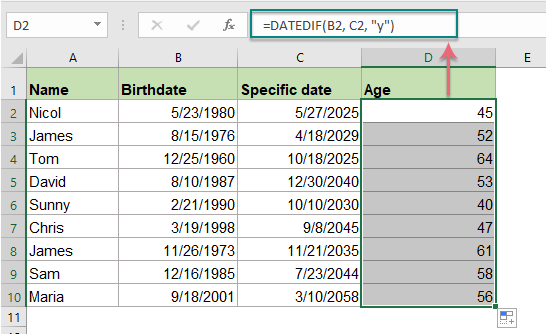Tips: To get the exact years, months and days of the age, please use the below formula:

=DATEDIF(B2, C2,"Y") & " Years, "& DATEDIF(B2,C2,"YM") & " Months, "&DATEDIF(B2,C2, "MD") & " Days"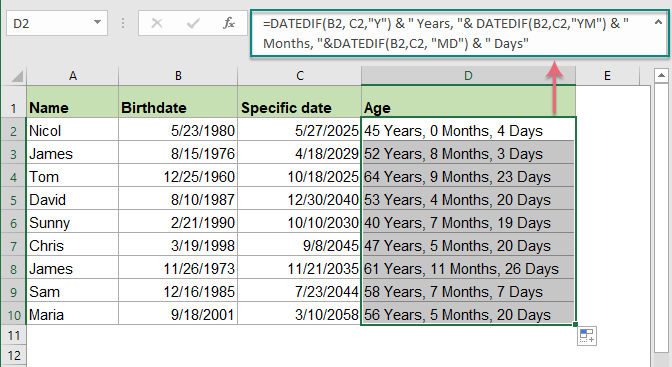#### Calculate age based on the birth of date before 1900

The above formulas don’t work correctly when the birth date is before 1900, because when entering a date prior to 1900, it will be stored as text format automatically in Excel. So, here is a User Defined Function helps you to get the age if the birth date is earlier than 1900.

1. Press the Alt + F11 keys to open the Microsoft Visual Basic for Applications window.

2. And then click Insert > Module, then copy the following VBA code into the Module window.

Calculate age from the birth of date before 1900 to today:

``````Function AgelnNow(ByVal xDate As Variant)
Dim xIA As Integer
xIA = 0
On Error Resume Next
xIA = DateDiff("yyyy", xDate, Now())
If (Month(Now()) < Month(xDate)) Or (Month(xDate) = Month(Now())) Then
If (Day(Now()) < Day(xDate)) Then
xIA = xIA - 1
End If
End If
If xIA = -1 Then
AgelnNow = "Error"
Else
AgelnNow = xIA
End If
End Function
``````

3. Then enter the following formula into a blank cell: =AgelnNow(A2) (A2 is the birth date cell)

Then drag the fill handle down to the cells that you want to get the age, all ages from the birth date to today have been calculated, see screenshot: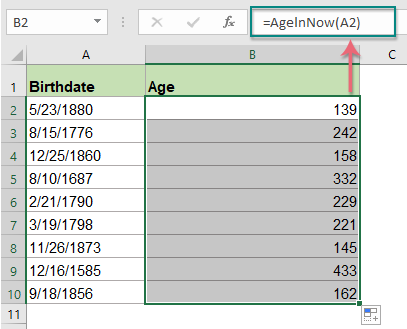Tip: If you need to get the age from birth date to death date instead of current date, please apply the below code:

Calculate age from the birth of date before 1900 to death date:

``````Function Age(ByVal StartDate As Variant, ByVal EndDate As Variant)
Dim xIA As Integer
xIA = 0
On Error Resume Next
xIA = DateDiff("yyyy", StartDate, EndDate)
If (Month(EndDate) < Month(StartDate)) Or (Month(StartDate) = Month(EndDate)) Then
If (Day(EndDate) < Day(StartDate)) Then
xIA = xIA - 1
End If
End If
If xIA = -1 Then
Age = "Error"
Else
Age = xIA
End If
End Function
``````

And use this formula: =Age(A2,B2) (A2 is the birth date cell, and B2 is the death date cell) to get the result as below screenshot shown: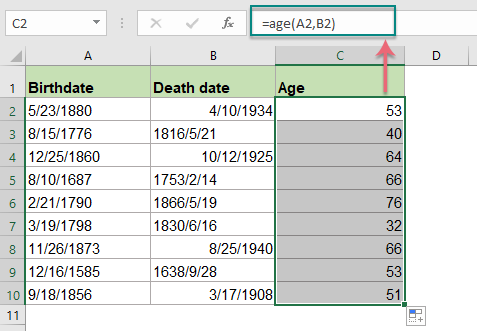#### Calculate age based on the birth of date from current or a specific date with an amazing feature

Kutools for Excel supports a handy tool - Date & Time Helper feature which can help you to calculate the age based on the birth of date from current date or a specific date as you need without remebering any painful formulas. Click to download Kutools for Excel for free trial!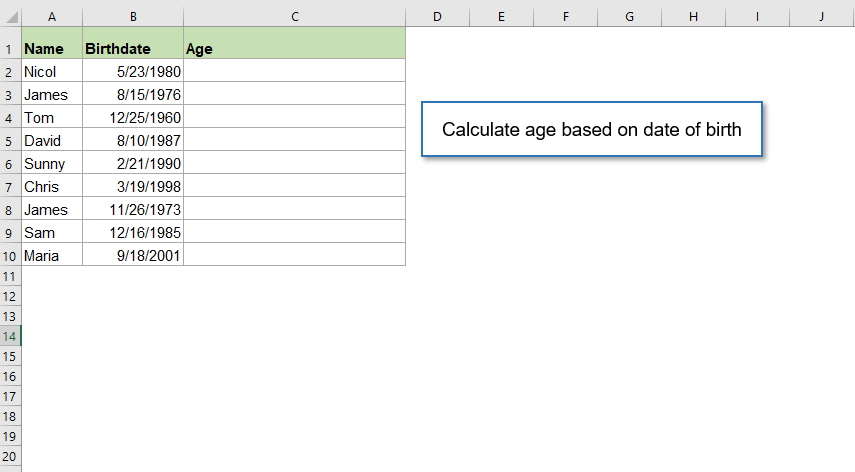#### Relative functions used:

• YEARFRAC:
• It returns a decimal value that represents fractional years between two dates.
• TODAY()
• It gives the current date value.
• INT()
• It returns the integer part of a value.
• DATEDIF
• This function returns the number of years, months, or days between two given dates.

#### Relative age calculation articles:

• Convert Birthdate To Age Quickly In Excel
• For instance, you get a range of various birthdate data in Excel, and you need to convert the birthdate to display their exact age value in Excel, how would you like to figure out?
• Calculate Age From ID Number In Excel
• Supposing, you have a list of ID numbers which contain 13 digit numbers, and the first 6 numbers is the birth date. For example, the ID number 9808020181286 means the birth date is 1998/08/02.

### The Best Office Productivity Tools

#### Kutools for Excel - Helps You To Stand Out From Crowd

 Popular Features: Find, Highlight or Identify Duplicates  |  Delete Blank Rows  |  Combine Columns or Cells without Losing Data  |  Round without Formula ... Super VLookup: Multiple Criteria  |  Multiple Value  |  Across Multi-Sheets  |  Fuzzy Lookup... Adv. Drop-down List: Easy Drop Down List  |  Dependent Drop Down List  |  Multi-select Drop Down List... Column Manager: Add a Specific Number of Columns  |  Move Columns  |  Toggle Visibility Status of Hidden Columns  |  Compare Columns to Select Same & Different Cells ... Featured Features: Grid Focus  |  Design View  |  Big Formula Bar  |  Workbook & Sheet Manager | Resource Library (Auto Text)  |  Date Picker  |  Combine Worksheets  |  Encrypt/Decrypt Cells  |  Send Emails by List  |  Super Filter  |  Special Filter (filter bold/italic/strikethrough...) ... Top 15 Toolsets:  12 Text Tools (Add Text, Remove Characters ...)  |  50+ Chart Types (Gantt Chart ...)  |  40+ Practical Formulas (Calculate age based on birthday ...)  |  19 Insertion Tools (Insert QR Code, Insert Picture from Path ...)  |  12 Conversion Tools (Numbers to Words, Currency Conversion ...)  |  7 Merge & Split Tools (Advanced Combine Rows, Split Excel Cells ...)  |  ... and more

Kutools for Excel Boasts Over 300 Features, Ensuring That What You Need is Just A Click Away...#### Office Tab - Enable Tabbed Reading and Editing in Microsoft Office (include Excel)

• One second to switch between dozens of open documents!
• Reduce hundreds of mouse clicks for you every day, say goodbye to mouse hand.
• Increases your productivity by 50% when viewing and editing multiple documents.
• Brings Efficient Tabs to Office (include Excel), Just Like Chrome, Edge and Firefox.